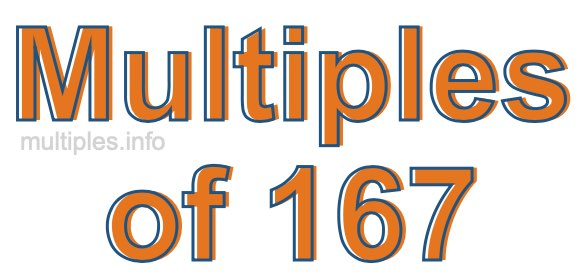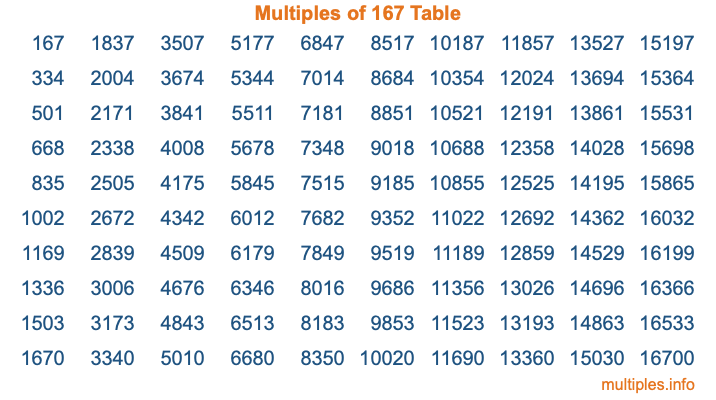Multiples of 167Welcome to the Multiples of 167 page. Here we will first teach you everything you will ever need to know about the multiples of 167, and then give you a study guide summary of everything we taught you to make sure you remember it all. Use this page to look up facts and learn information about the multiples of 167. This page will make you a multiples of one hundred sixty-seven expert!

Definition of Multiples of 167
Multiples of 167 are all the numbers that when divided by 167 equal an integer. Each of the multiples of 167 are called a multiple. A multiple of 167 is created by multiplying 167 by an integer.

Therefore, to create a list of multiples of 167, you start with 1 multiplied by 167, then 2 multiplied by 167, then 3 multiplied by 167, and so on for as long as you want. Thus, the list of the first five multiples of 167 is 167, 334, 501, 668, and 835. To see a larger list of multiples of 167, see the printable image of Multiples of 167 further down on this page. We also have a category where you can choose any nth multiple of 167.

Multiples of 167 Checker
The Multiples of 167 Checker below checks to see if any number of your choice is a multiple of 167. In other words, it checks to see if there is any number (integer) that when multiplied by 167 will equal your number. To do that, we divide your number by 167. If the the quotient is an integer, then your number is a multiple of 167.

Is  a multiple of 167?

Least Common Multiple of 167 and ...
A Least Common Multiple (LCM) is the lowest multiple that two or more numbers have in common. This is also called the smallest common multiple or lowest common multiple and is useful to know when you are adding our subtracting fractions. Enter one or more numbers below (167 is already entered) to find the LCM.

Check out our LCM Calculator if you need more details about the Least Common Multiple or if you need the LCM for different numbers for adding and subtraction fractions.

nth Multiple of 167
As we stated above, 167 is the first multiple of 167, 334 is the second multiple of 167, 501 is the third multiple of 167, and so on. Enter a number below to find the nth multiple of 167.

th multiple of 167

Multiples of 167 vs Factors of 167
167 is a multiple of 167 and a factor of 167, but that is where the similarities end. All postive multiples of 167 are 167 or greater than 167. All positive factors of 167 are 167 or less than 167.

Below is the beginning list of multiples of 167 and the factors of 167 so you can compare:

Multiples of 167: 167, 334, 501, 668, 835, etc.

Factors of 167: 1, 167

As you can see, the multiples of 167 are all the numbers that you can divide by 167 to get a whole number. The factors of 167, on the other hand, are all the whole numbers that you can multiply by another whole number to get 167.

It's also interesting to note that if a number (x) is a factor of 167, then 167 will also be a multiple of that number (x).

Multiples of 167 vs Divisors of 167
The divisors of 167 are all the integers that 167 can be divided by evenly. Below is a list of the divisors of 167.

Divisors of 167: 1, 167

The interesting thing to note here is that if you take any multiple of 167 and divide it by a divisor of 167, you will see that the quotient is an integer.

Multiples of 167 Table
Below is an image of the first 100 multiples of 167 in a table. The table is in chronological order, column by column. The first column has the first ten multiples of 167, the second column has the next ten multiples of 167, and so on.The Multiples of 167 Table is also referred to as the 167 Times Table or Times Table of 167. You are welcome to print out our table for your studies.

Negative Multiples of 167
Although not often discussed or needed in math, it is worth mentioning that you can make a list of negative multiples of 167 by multiplying 167 by -1, then by -2, then by -3, and so on, to get the following list of negative multiples of 167:

-167, -334, -501, -668, -835, etc.

Multiples of 167 Summary
Below is a summary of important Multiples of 167 facts that we have discussed on this page. To retain the knowledge on this page, we recommend that you read through the summary and explain to yourself or a study partner why they hold true.

There are an infinite number of multiples of 167.

A multiple of 167 divided by 167 will equal a whole number.

167 divided by a factor of 167 equals a divisor of 167.

The nth multiple of 167 is n times 167.

The largest factor of 167 is equal to the first positive multiple of 167.

167 is a multiple of every factor of 167.

167 is a multiple of 167.

A multiple of 167 divided by a divisor of 167 equals an integer.

167 divided by a divisor of 167 equals a factor of 167.

Any integer times 167 will equal a multiple of 167.

Multiples of a Number
Here you can get the multiples of another number, all with the same attention to detail as we did for multiples of 167 on this page.

Multiples of
Multiples of 168
Did you find our page about multiples of one hundred sixty-seven educational? Do you want more knowledge? Check out the multiples of the next number on our list!